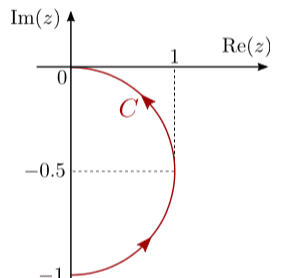# Contour Integrals: Working Check

WWCY

## Homework Statement

Hi all, could someone help me run through my work for these 2 integrals and see if I'm in the right direction? I'm feeling rather unsure of my work.

1) Evaluate ##\oint _\Gamma Z^*dz## along an anticlockwise circle of radius R centered at z = 0

2) Calculate the contour integral ##\int _C z^n dz## where n ∈ N, and C is a semi-circle contour as shown:## The Attempt at a Solution

1)
The contour is parameterized by ##Z = Re^{i\theta}##, with ##0 <\theta < 2\pi##. Therefore:

##\oint _\Gamma Z^*dz = \int_{\theta _ 1}^{\theta _2} d\theta \frac{dz}{d\theta} f(z(\theta))## and,

##\int_{\theta _ 1}^{\theta _2} d\theta ( Rie^{i\theta}) (Re^{-i\theta})## thus giving

##R^2i\int_{\theta _ 1}^{\theta _2} d\theta = 2\pi R^2 i##

2)

I define another integral ##I' = \oint_\Gamma Z^n dz## where ##\Gamma## forms a closed loop of the semi-circle with original contour C and an additional contour from 0i to -i that I define as C'

I then have,

##I' = \oint_\Gamma Z^n dz = \int_{C'} Z^n dz + \int_{C}Z^n dz ##

According to Cauchy's integral theorem, ##I'## on the whole should give 0 as ##Z^n## is analytic everywhere in ##\Gamma##, leaving me with

##- \int_{C'} Z^n dz = \int_{C}Z^n dz ##

C' is parameterized by ##Z = it## with ##t## ranging from 0 to -1, hence

##- \int_{C'} Z^n dz = \int_{0}^{-1} dt \frac{dz}{dt} f(z(t))##

##- \int_{C'} Z^n dz = \int_{0}^{-1} dt(i)(it^n)##

## \int_{0}^{-1} dt(i)(it^n) = i^{n+1} \int_{0}^{-1} t^n dt = (i^{n+1})[\frac{-1^{n+1}}{n+1}]##

## \int_{C}Z^n dz = - \int_{C'} Z^n dz = (-1) (i^{n+1})[\frac{-1^{n+1}}{n+1}] = (i^{n+1})[\frac{-1^{n+2}}{n+1}]##

this then gives 2 solutions for odd and even ##n##.

## \int_{C}Z^n dz = \frac{-i^{n+1}}{n+1}## for odd ##n##

## \int_{C}Z^n dz = \frac{i^{n+1}}{n+1}## for even ##n##

Help is greatly appreciated!

#### Attachments

Staff Emeritus
Homework Helper
Gold Member
It looks mostly fine, but you really should not write ##(-1)^k## as ##-1^k##. With correct order of operations, exponentiation comes before subtraction and so ##-1^k = -1##.

Also, instead of writing your result as separate expressions for odd and even ##n##, I suggest using that ##-1 = i^{-2}## in order to simplify your expression significantly.

WWCY
Also, instead of writing your result as separate expressions for odd and even ##n##, I suggest using that ##-1 = i^{-2}## in order to simplify your expression significantly.

Hi @Orodruin, thanks for assisting, I will make the necessary adjustments.

With regards to the quote above, I realized that I could re-write the odd n solution as ##\frac{i^{n-1}}{n+1}##, but I can't see how I can combine the answers as per the suggestion. Do you mind elaborating? Thank you!

Staff Emeritus
Homework Helper
Gold Member

##(i^{n+1})[\frac{(-1)^{n+2}}{n+1}]##

and use ##-1 = i^{-2}## instead of trying to start from the separated expressions.

WWCY

and use ##-1 = i^{-2}## instead of trying to start from the separated expressions.

This gives ##\frac{i^{-(n+1)}}{n+1}## for all n, is that right?

Staff Emeritus
Homework Helper
Gold Member
You should check your computation by checking if it holds for some particular ##n##. Try it for ##n = 0##.

WWCY
Whoops, made a ridiculous error. I believe it should've been ##\frac{i^{-(n+3)}}{n+1} ## instead, is that right?

Staff Emeritus